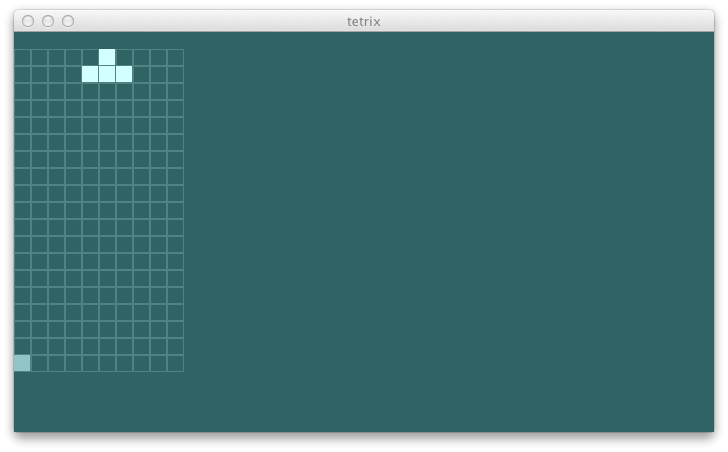### drawing the game

This is enough information to start drawing the game better.

``````  def onPaint(g: Graphics2D) {
val view = ui.view

def buildRect(pos: (Int, Int)): Rectangle =
new Rectangle(pos._1 * (blockSize + blockMargin),
(view.gridSize._2 - pos._2 - 1) * (blockSize + blockMargin),
blockSize, blockSize)
def drawEmptyGrid {
g setColor bluishLigherGray
for {
x <- 0 to view.gridSize._1 - 1
y <- 0 to view.gridSize._2 - 2
val pos = (x, y)
} g draw buildRect(pos)
}
def drawBlocks {
g setColor bluishEvenLigher
view.blocks foreach { b => g fill buildRect(b.pos) }
}
def drawCurrent {
g setColor bluishSilver
view.current foreach { b => g fill buildRect(b.pos) }
}
drawEmptyGrid
drawBlocks
drawCurrent
}
``````

Now that we have a way to visualize the game state, we should implement some moves.

### stage

We need a better way of representing the current piece besides a sequence of blocks. A `Piece` class should keep the current position in `(Double, Double)` and calculate the `current` from the local coordinate system.

``````case class Piece(pos: (Double, Double), kind: PieceKind, locals: Seq[(Double, Double)]) {
def current: Seq[Block] =
locals map { case (x, y) =>
Block((math.floor(x + pos._1).toInt, math.floor(y + pos._2).toInt), kind)
}
}
case object Piece {
def apply(pos: (Double, Double), kind: PieceKind): Piece =
kind match {
case TKind => Piece(pos, kind, Seq((-1.0, 0.0), (0.0, 0.0), (1.0, 0.0), (0.0, 1.0)))
}
}
``````

This allows us to define `Stage` which enforces the physics within the game world.

``````package com.eed3si9n.tetrix

class Stage(size: (Int, Int)) {
private[this] def dropOffPos = (size._1 / 2.0, size._2 - 3.0)
private[this] var currentPiece = Piece(dropOffPos, TKind)
private[this] var blocks = Block((0, 0), TKind) +: currentPiece.current
def view: GameView = GameView(blocks, size, currentPiece.current)
}
``````

To move the current piece, it is unloaded from the grid, moved to a new position, and then reloaded back in.

Here’s the `moveBy` for `Piece` class:

``````  def moveBy(delta: (Double, Double)): Piece =
copy(pos = (pos._1 + delta._1, pos._2 + delta._2))
``````

``````class Stage(size: (Int, Int)) {
...

def moveLeft() = moveBy(-1.0, 0.0)
def moveRight() = moveBy(1.0, 0.0)
private[this] def moveBy(delta: (Double, Double)): this.type = {
val moved = currentPiece.moveBy(delta)
currentPiece = moved
this
}
private[this] def unload(p: Piece, bs: Seq[Block]): Seq[Block] = {
val currentPoss = p.current map {_.pos}
bs filterNot { currentPoss contains _.pos  }
}
private[this] def load(p: Piece, bs: Seq[Block]): Seq[Block] =
bs ++ p.current
}
``````

### wiring it up

Let’s wire the stage up to the abstract UI:

``````package com.eed3si9n.tetrix

class AbstractUI {
private[this] val stage = new Stage((10, 20))
def left() {
stage.moveLeft()
}
def right() {
stage.moveRight()
}
def up() {
}
def down() {
}
def space() {
}
def view: GameView = stage.view
}
``````

You should be able run swing UI and see the piece move.